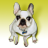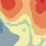# What are the weights in the semi-variogram map in Geostatistical wizard?

964
12
09-10-2013 01:59 PMNew Contributor
Hi,

If you generate the Semivariogram using Geostatistical wizard. it will show you the Semivariogram map at the bottom of the window. (which looks like a raster map) (following image)

[ATTACH=CONFIG]27332[/ATTACH]

when you move the mouse pointer on the map, two numbers will pop up for each pixel
one is the Value of the cell and the other one is the Weight.

I understand that the "Value" should be the average of moment of inertia of the pairs in that specific bin
But I don't know what is the "Weight"? I could not find anything regarding to this number in the manual.

I would appreciate if someone could shed some light on this for me.

Regards

E
Tags (3)
1 Solution

Accepted SolutionsbyEsri Regular Contributor

Here's a quick test: make two points with the same coordinates so that their vector plots at the origin.  The (1,1) and (1,-1) bins should each get a weight of 0.5 (they'll each get 0.25 naturally, then another 0.25 from the inflection).

If that doesn't work, I don't think I can help you.  You've pretty much exhausted my knowledge of our binning mechanism.
12 RepliesbyEsri Regular Contributor
You can find a full description of binning mechanism and weights in this paper:

When you hover over a cell in the semivariogram map, the weight you see is the number of pairs of points that fall within that bin.  The reason the weights aren't whole numbers is that the individual weight of each pair is distributed into the surrounding bins.  For example, if a pair of points falls exactly on the corner between 4 square bins, each of those bins will get a weight of 0.25 from that pair.  If the pair falls exactly at the center of a square bin, that bin will get a full weight of 1, and all other bins will get 0 weight.  The "Weight" in the semivariogram map is the sum of all weights for all pairs of points within that bin.New Contributor
Thank you very much.

This is exactly what I was looking for.

ENew Contributor
Hi

I went through the paper and I am able to generate exactly the same numbers for the semivariogram map cells (bins) manually.
If the cells are not located on the boundaries, or close the axes.

There is no description of how program handles the calculation of the boundary cells in the paper.
I wonder if you guys could please point me toward more detailed paper.

Also I have another question. after Geospatial wizard calculated the bin values, it somehow averages them and then fits a theoretical curve to the average values.

How does it average the bins?
I couldn't find it in the paper. So I would appreciate if you could reply to these questions as well.

Regards,

EhsanbyEsri Regular Contributor
Regarding the boundary cells, the description is on page 6.  Also, there's a picture of the triangular kernels in Figure 6 (in the appendix).

And the averaging of the bins is the average of the semivariances of all pairs within that bin.  The trick is that there are actually two binning mechanisms: one involves gridded cells and the other uses concentric circles (Figures 2 and 3 in the appendix).  In the semivariogram UI, the red dots come from the gridded cells, and the blue crosses come from the concentric circles.  These circular bins are what are actually used to fit the semivariogram (the red dots are more for diagnostic information and for calculating the semivariogram map).  The semivariogram parameters are estimated using weighted least-squares, where the weights come from the (modified) number of points that fall within each circular bin.New Contributor
Thanks Eric for your quick response.

I have seen the method in which the pairs were weighted on the cell (bin) boundaries and I used it to manually calculate the bin value but from the boundary cells, what I meant is the cells (bins) which are located close to the center axe lines of the SV map.
(i.e. SV map is drawn on a Cartesian axes, the center of these axes are located in the center of the map circle)

Cells on these boundary bins have a slightly different value. that I am not sure where it comes from

I got the answer for my second question. thanks

ebyEsri Regular Contributor
Remember that the vector between two points, A and B, can be defined two ways: from A to B and from B to A.  For this reason, the bins in the 1st and 3rd quadrants are identical, and so are the bins in the 2nd and 4th quadrants.  This is why the semivariogram map is symmetrical about the two diagonals.

Keeping this in mind, you need to pick two quadrants to use (we use the 1st and 2nd), then you'll need to inflect any weights that fall into the 3rd and 4th quadrants.  Be careful not to double-count each pair.New Contributor
Eric,

Thanks for your response and great help
I think there is a small miscommunication here.

I understand the way the method works and I have calculated the values and the weights for half of the pairs then reflected them based on the center point to create the full map.

If you look at the following image it might help to better understand the issue.

[ATTACH=CONFIG]27405[/ATTACH]

Let's just consider the first quadrant (upper-right)

I am able to calculate the cell values and weights for all of the cells which are located under the Blue rectangle. and I get the same number as shown in the map. so no problem with these cells.

That being said, when I use the same method on the cells which are located under the red rectangles my calculated values and weights are slightly less than what is shown on the map.

My understanding is that these cells (under red rec.) have smaller coverage area in comparison with other cells because they are limited to the axial lines

So I hope this further explain the issue.

Regards,
ebyEsri Regular Contributor
I talked this over with the developer that programmed the semivariogram functions, and we're not sure why your red bands aren't matching ours.  We suspect that you have a bug in the part of the algorithm that inflects weights that cross over the axes, but we're not sure.

When you're inflecting the weights, make sure that you are rotating them rather than just mirroring them.  That could be the problem.New Contributor
I am not sure what do you mean by rotating.

Here is what I have done.

I calculate the moment of inertia for all the pairs that look toward right. (Xj>=Xi)
this will give me the n(n-1)/2 pairs out of n point.

then I find all the pairs located in each bin and its shadows
problem is that in the red band bins, the shadow of each bin is smaller than the blue band bins

The concept could be better understood by the following shape

[ATTACH=CONFIG]27406[/ATTACH]

Let's consider the (1,1) bin (the corner bin in the first quadrant).
only three edges of this bin have shadows so the area that it covers is less than the blue band bins which have full shadow area.

then to calculate the bin values for third quadrant (lower-left)
I just multiply the coordinates of the center of each bins to -1 and copy the weights and values.

I think there should be some sort of modification for calculating the red band values on your side that is not explained in the paper.

Thanks for you time and kind attention

e